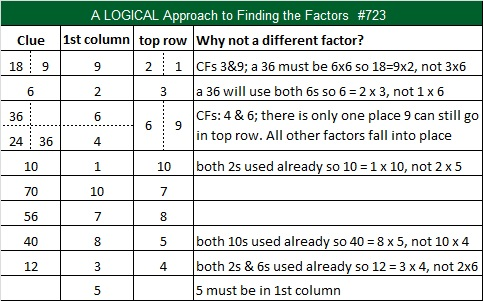# 723 and Level 6

7 + 2 + 3 = 12, a multiple of 3, so 723 is divisible by 3.

• 723 is a composite number.
• Prime factorization: 723 = 3 x 241
• The exponents in the prime factorization are 1 and 1. Adding one to each and multiplying we get (1 + 1)(1 + 1) = 2 x 2 = 4. Therefore 723 has exactly 4 factors.
• Factors of 723: 1, 3, 241, 723
• Factor pairs: 723 = 1 x 723 or 3 x 241
• 723 has no square factors that allow its square root to be simplified. √723 ≈ 26.88866.Finding the factors to solve this Level 6 puzzle will be more challenging than that. . .Print the puzzles or type the solution on this excel file: 10 Factors 2015-12-21

—————————————————————————————————

Here is a little more about the number 723:

723 is the sum of consecutive numbers three different ways:

• 361 + 362 = 723; that’s 2 consecutive numbers.
• 240 + 241 + 242 = 723; that’s 3 consecutive numbers.
• 118 + 119 + 120 + 121 + 122 + 123 = 723; that’s 6 consecutive numbers.

Because 241 is one of its factors, 723 is the hypotenuse of the Pythagorean triple 360-627-723. What is the greatest common factor of those three numbers? (It is one of the four factors of 723.)

723 is repdigit and palindrome 333 in BASE 15; note that 3(15²) + 3(15) + 3(1) = 723.

Stetson.edu informs us that  (1!)! + (2!)! + (3!)! = 723. That looks more exciting than it really is. It is only equivalent to saying 1 + 2 + 720 = 723.

—————————————————————————————————Thank you, Ricardo, for completing the puzzle and tweeting your work: